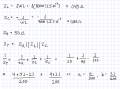# Is my solution or the solutions manual incorrect?

#### Aria Nemati

Joined Jul 19, 2019
27

#### Papabravo

Joined Feb 24, 2006
17,299
Your solution is wrong. It should be:
$Y_C\;=\;\frac{1}{Z_C}\;=\;\frac{1}{\frac{1}{j\omega C}}\;=\;j\omega C$

#### Aria Nemati

Joined Jul 19, 2019
27
But Zc is negative. Where does the negative sign go?

#### Papabravo

Joined Feb 24, 2006
17,299
But Zc is negative. Where does the negative sign go?
When you take the reciprocal it disappears.
$\frac{1}{j}\;=\; -j$
Right?

#### Aria Nemati

Joined Jul 19, 2019
27
When you take the reciprocal it disappears.
$\frac{1}{j}\;=\; -j$
Right?
Ok. I'm getting confused now. I just did a problem that is almost the same and got the correct answer. So what is the difference that I have failed to see?Now I did the same problem the way I am supposed to and I get a wrong answer:The angle is wrong. It should be positive not negative. So what am I doing wrong here?

Last edited:

#### The Electrician

Joined Oct 9, 2007
2,866
How do you know what the correct solutions for post #5 and #6 are? Are those also worked out in a solutions manual? If so, post the solutions given in the solutions manual.